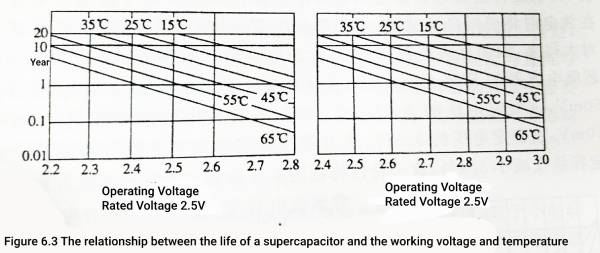Contents

Supercapacitor, also known as ultracapacitors or electrochemical capacitors, are energy storage devices that have higher power density and faster charging and discharging rates than conventional batteries.The supercapacitor voltage index mainly include rated DC voltage, working voltage, rated surge voltage and decomposition voltage, which will be introduced one by one below.

## 1 Supercapacitor Voltage-Rated DC voltage Vr

The rated DC voltage Vr is the continuous working voltage allowed by the capacitor within the rated temperature range, which includes the sum of the DC voltage and the pulse voltage or continuous pulse voltage between the two electrodes of the capacitor. Different from other capacitors, the life of supercapacitors decreases with the increase of working voltage, so the rated working voltage is actually the voltage value on the basis of ensuring the life of supercapacitors. Usually, the rated voltage of the supercapacitor is marked on the surface of the capacitor. At present, supercapacitors are divided into two types: water system and organic according to different electrolytes: the rated voltage of the water system supercapacitor is 1.4V or the same voltage level as the nickel metal hydride battery, and the organic system is 2.3V, 2.5V, 2.7V .

## 2 Supercapacitor Voltage-Working voltage Vop

The working voltage is the voltage that the capacitor is allowed to work continuously within the rated temperature range. In the entire operating temperature range, the capacitor can work continuously at the full rated voltage (including AC superimposed voltage), or at any voltage value between 0V and the rated voltage.

In fact, because the capacitance of the supercapacitor is too large, a very large current will flow even if there is a little AC superimposed voltage. In fact, the AC voltage that can be applied to the supercapacitor is limited by the current that the supercapacitor can withstand. For example, a 3000F supercapacitor can pass a peak current of 2000A, according to the relationship between the current and voltage of the capacitor

dv/dt=I/C=2000/3000=2/3(V/s)=2*10-6/3（V/s）

For AC 220V, the corresponding frequency will be microhertz level, which is DC voltage. Therefore, the so-called AC superimposed voltage can be ignored, and it is not necessary to study the AC rated voltage of supercapacitors.

## 3 Rated surge voltage Vs

Rated surge voltage Vs is the short-term voltage value that aluminum electrolytic capacitors can withstand. The test conditions are: the capacitor works at 25°C and does not exceed 30s, and the interval between two surges is not less than 5min. Rated voltages are 2.3, 2.5, 2.7V. The rated surge voltages of supercapacitors are 2.7V, 2.8V and 2.85V respectively, which are about 1.1 times the rated voltage. It can be seen from the rated surge voltage value that the surge voltage tends to 2.85V, that is to say, no matter whether the rated voltage is 2.3V, 2.5V or 2.7V, the surge voltage value does not change much, which shows that the supercapacitor’s The rated voltage can be set at 2.7V. The rated voltages of 2.3V, 2.5V, and 2.7V are actually caused by differences in the manufacturing level of supercapacitors in different periods. This can be explained from the age of each rated voltage. For example, 2.3V is usually a product developed before 1999, 2.5V is a product developed in 2000 and 2001, and 2.7V is a product developed around 2002. , Some companies claim to launch 3V class supercapacitors in 2003, but there are still no commercial products. This shows that by improving the manufacturing process of supercapacitors and improving the quality of raw materials, the performance of supercapacitors can be improved without a revolution in materials, and the withstand voltage will not be greatly improved.

## 4 Decomposition voltage

The decomposition voltage is the limit voltage that the “electrolyte” of the supercapacitor can withstand. Once the general capacitor dielectric exceeds the dielectric strength of the medium, it will be broken down or even become a conductor. Once the medium (“electrolyte”) in a supercapacitor exceeds the redox electrode potential (“dielectric strength”) of the electrolyte, the electrolyte will be decomposed into other substances (such as water and some gases), and the stored energy will be released. Electrolyte” decomposition and temperature rise absorbed.
The decomposition voltage of the current aqueous supercapacitor is not lower than the voltage level of the nickel-metal hydride battery; that is, it does not exceed 1.6V; the decomposition voltage of the organic series supercapacitor is at least not lower than 3.6V. The ratio to the rated voltage is about 1.3~1.5 times

## 5 Relationship between working voltage and life

The electrolyte of the supercapacitor increases with the temperature and the molecular motion speed increases, so that the volatilization speed of the electrolyte increases with the temperature, and the volatilization or decomposition speed of the electrolyte also increases with the increase of the applied voltage of the supercapacitor, which is different from other capacitors. different. Because of this, it is better for supercapacitors not to apply voltage when they are not working or stored, which is just the opposite of aluminum electrolytic capacitors.
The influence of operating voltage and temperature on life of supercapacitors is shown in Figure 6.3. It can be seen from the figure that under the same temperature conditions, the working voltage increases by 0.1V, and the life span is halved. Therefore, appropriately reducing the operating voltage is beneficial to the life of supercapacitors.